# smoothr: Smooth and Tidy Spatial Features in R

smoothr offers a variety of tools for smoothing and tidying spatial features (i.e. polygons and lines) to make them more aesthetically pleasing, especially when converting raster data to vector format. This package offers support for both sp and sf spatial objects. The following smoothing methods are available:

• Chaikin’s corner cutting algorithm: smoothing using Chaikin’s corner cutting algorithm, which iteratively replaces every point by two new points: one 1/4 of the way to the next point and one 1/4 of the way to the previous point. This method applies a moderate amount of smoothing of sharp corners without extensive generalization.
• Kernel smoothing: smoothing using Gaussian kernel regression via the ksmooth() function. This approach first densifies the feature (i.e. adds more vertices) then applies the kernel smoothing. Kernel smoothing simultaneously smooths and generalizes curves, and can be turned to produce extensively smoothed curves.
• Spline interpolation: smoothing using spline interpolation via the spline() function. This method interpolates between existing vertices and should be used when the resulting smoothed feature must pass through the vertices of the input feature.

In addition to these smoothing functions, smoothr offers functions for filling polygon holes and dropping line and polygon fragments based on a size threshold, as well as densification (i.e. adding additional vertices along curves).

## Setup

library(raster)
library(sf)
library(units)
library(smoothr)

## Example data

This package comes with two simple spatial datasets in sf format to test the smoothing algorithms on. jagged_polygons contains 9 polygons with sharp corners begging to be smoothed out: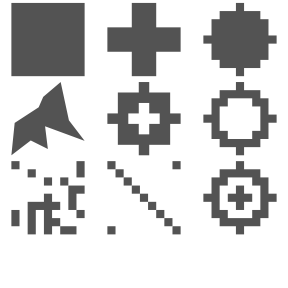Notice that these polygons have a range of complexities, some have holes, and some are mutlipart polygons. I’ve added a few flags to distinguish between the different types.

#> Simple feature collection with 9 features and 4 fields
#> geometry type:  GEOMETRY
#> dimension:      XY
#> bbox:           xmin: 0 ymin: 0 xmax: 1 ymax: 1
#> epsg (SRID):    4326
#> proj4string:    +proj=longlat +datum=WGS84 +no_defs
#>   id    type  hole multipart                       geometry
#> 1  1 polygon FALSE     FALSE POLYGON ((0.4444444 0.55555...
#> 2  2 polygon FALSE     FALSE POLYGON ((0.5555556 0.44444...
#> 3  3 polygon FALSE     FALSE POLYGON ((0.5555556 0.11111...
#> 4  4 polygon FALSE     FALSE POLYGON ((0.7854351 0.47743...
#> 5  5 polygon  TRUE     FALSE POLYGON ((0.5555556 0.22222...
#> 6  6 polygon  TRUE     FALSE POLYGON ((0.5555556 0.11111...
#> 7  7 polygon FALSE      TRUE MULTIPOLYGON (((0.6666667 0...
#> 8  8 polygon FALSE      TRUE MULTIPOLYGON (((0 0.1111111...
#> 9  9 polygon  TRUE      TRUE MULTIPOLYGON (((0.5555556 0...

jagged_lines contains 9 polylines with disgustingly crooked edges.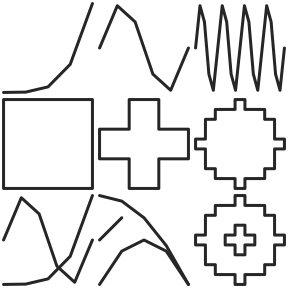Again, there’s a range of complexities, some lines form closed loops, and some are multipart.

#> Simple feature collection with 9 features and 4 fields
#> geometry type:  GEOMETRY
#> dimension:      XY
#> bbox:           xmin: 0 ymin: 0 xmax: 1 ymax: 1
#> epsg (SRID):    4326
#> proj4string:    +proj=longlat +datum=WGS84 +no_defs
#>   id type closed multipart                       geometry
#> 1  1 line  FALSE     FALSE LINESTRING (0 0, 0.25 0.003...
#> 2  2 line  FALSE     FALSE LINESTRING (0 0.5, 0.2 0.97...
#> 3  3 line  FALSE     FALSE LINESTRING (0 0.5, 0.05 0.9...
#> 4  4 line   TRUE     FALSE LINESTRING (0.4444444 0.555...
#> 5  5 line   TRUE     FALSE LINESTRING (0.5555556 0.444...
#> 6  6 line   TRUE     FALSE LINESTRING (0.5555556 0.111...
#> 7  7 line  FALSE      TRUE MULTILINESTRING ((0 0, 0.25...
#> 8  8 line  FALSE      TRUE MULTILINESTRING ((0 0, 0.25...
#> 9  9 line   TRUE      TRUE MULTILINESTRING ((0.5555556...

The final dataset that comes with this package, jagged_raster, is a simulated occurrence probability for a species, consisting of a spatially auto-correlated raster layer with values between 0 and 1. It is a 25x25 grid of 100 square kilometer cells in a North American centered Albers Equal Area projection. This raster can be used to experiment with smoothing polygons generated from rasters.

plot(rasterToPolygons(jagged_raster), col = NA, border = NA) # set up plot extent
plot(jagged_raster, col = heat.colors(100), legend = FALSE, add = TRUE)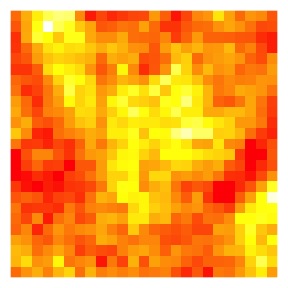## Smoothing methods

Currently, three smoothing methods have been implemented: Chaikin’s corner cutting algorithm, Gaussian kernel smoothing, and spline interpolation. All are accessed with the smooth() function, and all methods work on spatial lines and polygons in sf and sp format.

### Chaikin’s corner cutting algorithm

Chaikin’s corner cutting algorithm smooths by iteratively replacing every point by two new points: one 1/4 of the way to the next point and one 1/4 of the way to the previous point. This method applies a moderate amount of smoothing of sharp corners without extensive generalization, and is a good choice when the desire is to smooth without drastically altering the input features. In addition, this algorithm has the benefit of only requiring a single, easily interpretable parameter: the number of smoothing iterations.

This method can be applied with smooth(x, method = "chaikin"). Here’s what this looks like for the polygons:

p_smooth_chaikin <- smooth(jagged_polygons, method = "chaikin")
for (i in 1:nrow(jagged_polygons)) {
plot(st_geometry(jagged_polygons[i, ]), col = "grey40", border = NA)
plot(st_geometry(p_smooth_chaikin[i, ]), col = NA, border = "#E41A1C",
lwd = 2, add = TRUE)
}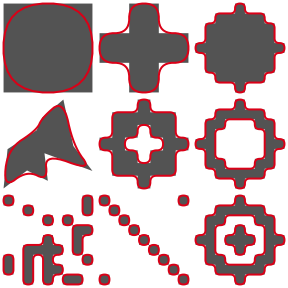And for the lines:

l_smooth_chaikin <- smooth(jagged_lines, method = "chaikin")
for (i in 1:nrow(jagged_lines)) {
plot(st_geometry(jagged_lines[i, ]), col = "grey20", lwd = 3)
plot(st_geometry(l_smooth_chaikin[i, ]), col = "#E41A1C", lwd = 2, add = TRUE)
}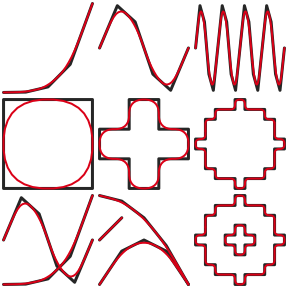### Kernel smoothing

This method applies Gaussian kernel regression to the x and y coordinates independently using the built-in ksmooth() function. Prior to smoothing, additional vertices are added via densification with smooth_densify(). For polygons (and closed lines), method = "periodic" is used to avoid getting a kink at the start/end of the curve defining the boundary.

Kernel smoothing simultaneously smooths and generalizes curves, and can be tuned to produce drastically smoothed curves. This method produces results similar to the PAEK algorithm used in ArcGIS. smooth_ksmooth() has parameters specifying the number of points in the resulting feature (either the number of sub-segments to split each line segment into or the maximum distance between points) and the bandwidth of the Gaussian kernel. Choosing a suitable bandwidth is critical for correctly smoothing features using this algorithm and typically users will want to explore a range of bandwidth to find a value that works for their particular scenario.

This method can be applied with smooth(x, method = "ksmooth"). Here’s what this looks like for the polygons:

p_smooth_ksmooth <- smooth(jagged_polygons, method = "ksmooth")
for (i in 1:nrow(jagged_polygons)) {
plot(st_geometry(jagged_polygons[i, ]), col = "grey40", border = NA)
plot(st_geometry(p_smooth_ksmooth[i, ]), col = NA, border = "#E41A1C",
lwd = 2, add = TRUE)
}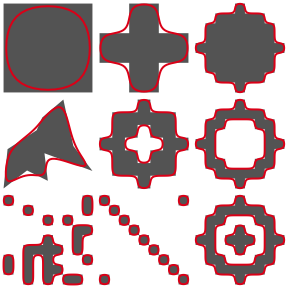And for the lines:

l_smooth_ksmooth <- smooth(jagged_lines, method = "ksmooth")
for (i in 1:nrow(jagged_lines)) {
plot(st_geometry(jagged_lines[i, ]), col = "grey20", lwd = 3)
plot(st_geometry(l_smooth_ksmooth[i, ]), col = "#E41A1C", lwd = 2, add = TRUE)
}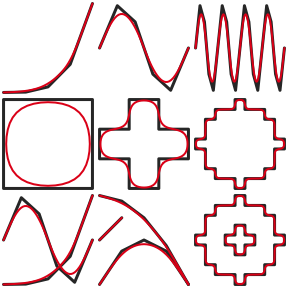### Spline interpolation

This method applies a spline interpolation to the x and y coordinates independently using the built-in spline() function. For polygons (and closed lines), method = "periodic" is used to avoid getting a kink at the start/end of the curve defining the boundary. Unlike the corner cutting algorithm, this method results in a curve that passes through the vertices of the original curve, which may be a desirable feature. Unfortunately, this results in an unnaturally wiggly curve. Spline interpolation requires a parameter specifying the number of points to interpolate at, which can either be an absolute number or a relative increase in the number of vertices.

This method can be applied with smooth(x, method = "spline"). Here’s what this looks like for the polygons:

p_smooth_spline <- smooth(jagged_polygons, method = "spline")
for (i in 1:nrow(jagged_polygons)) {
plot(st_geometry(p_smooth_spline[i, ]), col = NA, border = NA)
plot(st_geometry(jagged_polygons[i, ]), col = "grey40", border = NA,
plot(st_geometry(p_smooth_spline[i, ]), col = NA, border = "#E41A1C",
lwd = 2, add = TRUE)
}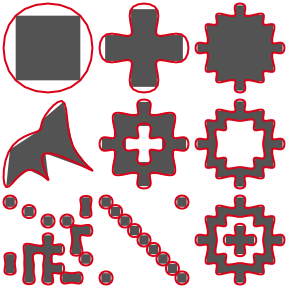And for the lines:

l_smooth_spline <- smooth(jagged_lines, method = "spline")
for (i in 1:nrow(jagged_lines)) {
plot(st_geometry(l_smooth_spline[i, ]), col = NA)
plot(st_geometry(jagged_lines[i, ]), col = "grey20", lwd = 3, add = TRUE)
plot(st_geometry(l_smooth_spline[i, ]), col = "#E41A1C", lwd = 2, add = TRUE)
}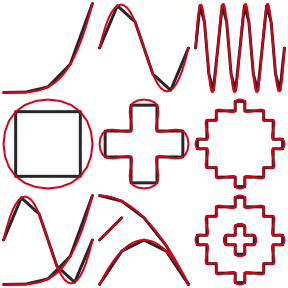## Other goodies

smoothr contains some additional tools that aren’t strictly for smoothing, but can help clean up polygons and lines and make them appear more aesthetically pleasing. I’ll outline these miscellaneous tools below.

### Densification

Densification is the process of adding additional points to the line segments defining polylines or polygons. Each line segment is split into multiple sub-segments of equal length, and the original vertices are always included in the densified feature. Note that the densification algorithm treats all vertices as Euclidean points, i.e. new points will not fall on a great circle between existing vertices, rather they’ll be along a straight line.

Use smooth(method = "densify"), or it’s alias densify() to perform densification. The degree of densification can either be specified as the number of sub-segments each line segment is split into (n):

l <- jagged_lines$geometry[] # split every segment into 2 l_dense <- densify(l, n = 2) plot(l, lwd = 5) plot(l_dense, col = "red", lwd = 2, lty = 2, add = TRUE) plot(l_dense %>% st_cast("MULTIPOINT"), col = "red", pch = 19, add = TRUE)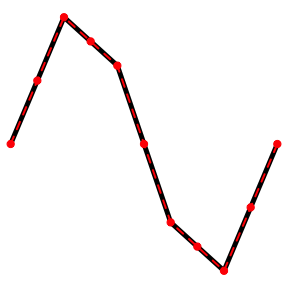Or the maximum Euclidean distance between vertices (max_distance): l <- jagged_lines$geometry[]
# split every segment into 2
l_dense <- densify(l, max_distance = 0.1)
plot(l, lwd = 5)
plot(l_dense, col = "red", lwd = 2, lty = 2, add = TRUE)
plot(l_dense %>% st_cast("MULTIPOINT"), col = "red", pch = 19, add = TRUE)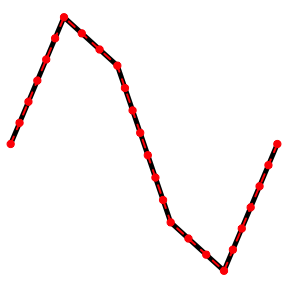### Drop crumbs

drop_crumbs() removes small lines or polygons based on a length or area threshold. For multipart features, this function dives into the individual component features and applies the threshold. The threshold can either be provided as a number, assumed to be in the units of the coordinates reference system (meters for unprojected coordinates), or as a units object. This latter approach provides more flexibility because a threshold can be given in any type of units and it will be converted to the correct units automatically.

For example, to remove polygons less than 200 square kilometers:

p <- jagged_polygons$geometry area_thresh <- units::set_units(200, km^2) p_dropped <- drop_crumbs(p, threshold = area_thresh) plot(p, col = "black", main = "Original") plot(p_dropped, col = "black", main = "After drop_crumbs()")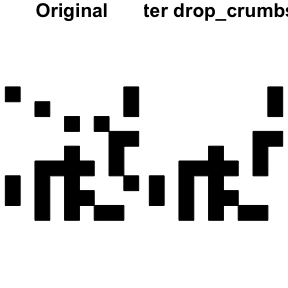And, to remove lines shorter than 25 miles: l <- jagged_lines$geometry
# note that any units can be used
# conversion to units of projection happens automatically
length_thresh <- units::set_units(25, miles)
l_dropped <- drop_crumbs(l, threshold = length_thresh)
plot(l, lwd = 5, main = "Original")
plot(l_dropped, lwd = 5, main = "After drop_crumbs()")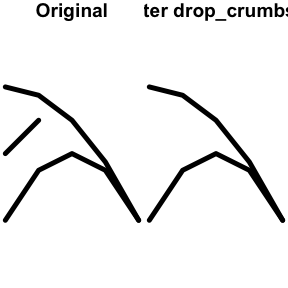### Fill holes

fill_holes() fills (i.e. removes) holes in polygons when they are below a given area threshold. As with drop_crumbs(), the threshold can either be provided as a number or a units object.

For example, to remove holes less than 800 square kilometers in size:

p <- jagged_polygons\$geometry
area_thresh <- units::set_units(800, km^2)
p_dropped <- fill_holes(p, threshold = area_thresh)
# plot
plot(p, col = "black", main = "Original")
plot(p_dropped, col = "black", main = "After fill_holes()")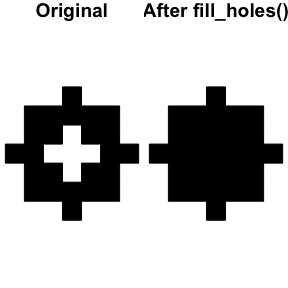## Raster-to-polygon conversion

The whole point of this smoothr business was to smooth out polygons generated from rasters, so let’s work through a quick example of that. Treating jagged_raster as the occurrence probability for a species, imagine we want to produce a range map for this species, showing where it occurs with at least 50% probability. We can convert the raster to a binary presence/absence map, then polygonize.

# pres/abs map
r <- cut(jagged_raster, breaks = c(-Inf, 0.5, Inf)) - 1
plot(rasterToPolygons(r), col = NA, border = NA) # set up plot extent
plot(r, col = c("white", "#4DAF4A"), legend = FALSE, add = TRUE, box = FALSE)
# polygonize
r_poly <- rasterToPolygons(r, function(x){x == 1}, dissolve = TRUE) %>%
st_as_sf()
plot(r_poly, col = NA, border = "grey20", lwd = 1.5, add = TRUE)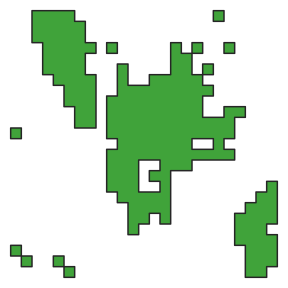To start cleaning this up, let’s remove all the small polygons resulting from a single raster cell. Recall that the cells is this raster are 100 square kilometers each.

r_poly_dropped <- drop_crumbs(r_poly, set_units(101, km^2))
# plot
plot(rasterToPolygons(r), col = NA, border = NA) # set up plot extent
plot(r_poly_dropped, col = "#4DAF4A", border = "grey20", lwd = 1.5, add = TRUE)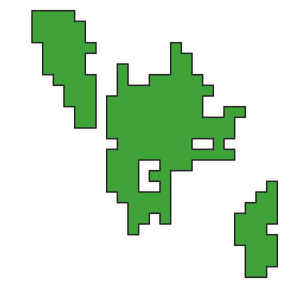There are two holes in the large central polygon. Next, let’s fill in the smaller of the two holes, which is two raster cells (200 square kilometers) in size, but keep the larger one.

r_poly_filled <- fill_holes(r_poly_dropped, set_units(201, km^2))
# plot
plot(rasterToPolygons(r), col = NA, border = NA) # set up plot extent
plot(r_poly_filled, col = "#4DAF4A", border = "grey20", lwd = 1.5, add = TRUE)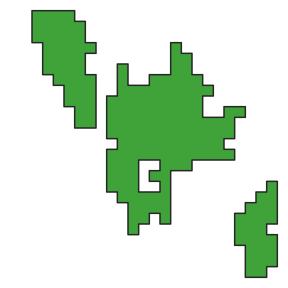Finally, to make this more aesthetically pleasing, I’ll smooth out those sharp edges.

r_poly_smooth <- smooth(r_poly_filled, method = "ksmooth")
# plot
plot(rasterToPolygons(r), col = NA, border = NA) # set up plot extent
plot(r_poly_smooth, col = "#4DAF4A", border = "grey20", lwd = 1.5, add = TRUE)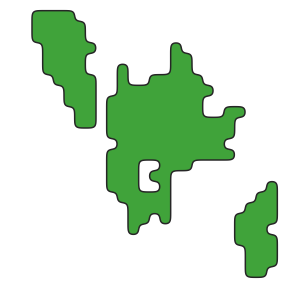For a greater degree of smoothing and generalization, increase the smoothness parameter:

r_poly_smooth <- smooth(r_poly_filled, method = "ksmooth", smoothness = 2)
# plot
plot(rasterToPolygons(r), col = NA, border = NA) # set up plot extent
plot(r_poly_smooth, col = "#4DAF4A", border = "grey20", lwd = 1.5, add = TRUE)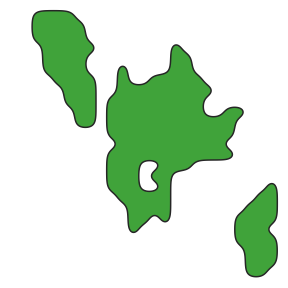Nice, so much easier on the eyes!